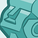# QlikView App Dev

Discussion Board for collaboration related to QlikView App Development.

Announcements
Welcome to Qlik Community! Check out our new navigation! FIND OUT MORE
cancel
Showing results for
Did you mean:Contributor III

## Using a ratio in the y-axis (Percentage) of a Scatter Chart.

Hello all,

I'm struggling with using a ratio percentage in the Y-axis. The ratio I'm using is the Contribution Margin Ratio. Essentially it is (Revenue - Cost) / Revenue

My end goal is to have an X-axis of Cost, Y-axis of contribution margin, and a Bubble size of overall Profitability. However, I can't seem to get a percentage for the Y-axis. I think I need to utilize Aggr, but I'm not quite sure. Here is my chart thus far.I would like the contribution margin ratio to be in place of the Revenue axis.

Here is the formula and variables I'm attempting to use.

Formula: \$(vf_OverallProfitability)/\$(vf_OverallRevenue)

Variables and definitions:

Profit (Total revenue minus the expenses)

\$(vf_OverallProfitability)

(sum({\$<[Resource Type] = {'Retained'}>} [#Hours Billed] * #Rate)

-sum({\$<[Resource Type] = {'Retained'}>} [#Consultant Retainer Amount Entered]))

+

(sum({\$<[Resource Type] = {'Employee'}>} [#Hours Worked] * #Rate)

-sum({\$<[Resource Type] = {'Employee'}>} [#Salary Amount Calculated]))

+

(sum({\$<[Resource Type] = {'Contractor'}>} [#Hours Worked] * #Rate)

-sum({\$<[Resource Type] = {'Contractor'}>} #Amount))

Revenue

\$(vf_OverallRevenue)

(sum({\$<[Resource Type] = {'Retained'}>} [#Hours Billed] * #Rate))

+

(sum({\$<[Resource Type] = {'Employee'}>} [#Hours Worked] * #Rate))

+

(sum({\$<[Resource Type] = {'Contractor'}>} [#Hours Worked] * #Rate))

When I use the above formula and variables in the graph I get whole numbers and my data points disappear from the graph.

Any help would be greatly appreciated here, I've been stuck on this problem for 2 days now.

Thanks,

Channing

1 Solution

Accepted SolutionsMVP

Maybe use extra brackets like

( \$(vf_OverallProfitability) ) / ( \$(vf_OverallRevenue) )

2 RepliesMVP

Maybe use extra brackets like

( \$(vf_OverallProfitability) ) / ( \$(vf_OverallRevenue) )Contributor III
Author

Feeling very silly now! Thank you